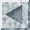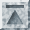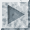In this chapter we discuss a few more advanced features of SST including the definition of functions and macros. SST allows users to design their own features which may include control loops and conditional operations. The final section of the chapter discusses the experimental graphics program included in SST. Currently SST supports high resolution scatterplots. It is anticipated that future releases will offer enhanced graphics capabilities.

## User defined functions

Frequently performed arithmetic operations can be speeded up by defining a function that performs the desired operations. For example, you may want to "standardize" a set of variables by deviating each from its mean and then dividing by its standard deviation. (The resulting variable has mean zero and variance one.) This operation could be accomplished repetively using `SET` statements:

```set z1 = (x1 - mean(x1))/stddev(x1)
set z2 = (x2 - mean(x2))/stddev(x2)
```

but this requires a lot of unnecessary typing. Instead, you could `DEFINE` a function `stand` to perform this operation:

```define stand(x) = (x - mean(x))/stddev(x)
```

The argument `x` to `stand` is a parameter that could be replaced with any variable name. The `SET` statements above can now be simplified to:

```set z1 = stand(x1)
set z2 = stand(x2)
```

SST functions can have many arguments. Each argument to a function should be separated by a comma. For example:

```define f(x,y,z) = (x - y)/z
```

To use the above function in a `SET` statement, you might try:

```set delprice = f(price2,price1,0.5)
```

The above example shows that constants (like 0.5) can be used as arguments to functions.

SST allows you to nest functions. That is, a function can be inserted in place of an argument to another function. For example:

```define yhat(b1,b2,b3) = x1*b1 + x2*b2 + x3*b3
define error(y1,y2) = y1-y2
set e = error(depvar,yhat(1.0,0.0,-1.0))
```

Thus, it is possible to build up rather complicated expressions. Any SST predefined function (e.g., `cumnorm` or `mean`) can also be used as an argument in a user-defined function.

To obtain a listing of functions defined in an SST sesssion, use the `LIST` command:

```list func
```

and SST will display the names and definitions of all functions that have been defined during the session. To inspect the definition of a one or more functions, add these as arguments to the `FUNC` subop:

```list func[stand]
```

and SST reproduces the definition for `stand`.

## Macro definitions

SST provides a macro facility that can substantially reduce the amount of typing required for entering commands, variable lists, and other user input. The simplest use of a macro is to define a variable list. Suppose for example that you expect to use the variables `pop15`, `pop75`, and `dpi` repeatedly in some subops. Define a variable list `vlist` using the `MACRO` command:

```macro vlist { pop15 pop75 dpi 2
```

Whenever you want to use this list of variables in a command, you just ask SST to expand the macro. For example:

```reg dep[sr] ind[one @vlist]
```

is equivalent to:

```reg dep[sr] ind[one pop15 pop75 dpi]
```

The @ symbol tells SST to expand the macro, i.e. to substitute the text of the macro in the command. Whenever you ask SST to expand a macro in a command, SST will display the expanded version of the command on the screen to remind you what the macro does.

More complicated macros may involve parameter lists and multiple commands. For example, it is possible to define a macro to perform two stage least squares. Suppose, for example, that we are interested in estimating an equations with one endogenous and two exogenous variables among the independent variables and that we have two additional exogenous variables to be used as instruments. In the first stage of two stage least squares, we regress the included endogenous variable on all the exogenous variables and save the predicted values. In the second stage we regress the dependent variable on the predicted values from the first stage regression and the included exogenous variables. To define a macro `2sls` which performs this operation in one shot, type:

```macro 2sls(y1,y2,x1,x2,z1,z2) {
reg dep[y2] ind[x1 x2 z1 z2] pred[y2hat]
reg dep[y1] ind[y2hat x1 x2] coef[b2sls]
set e2sls = y1 - b2sls(1)*y2 - b2sls(2)*x1 - b2sls(3)*x2
calc stdev(e2sls)
}
```

The last two commands are necessary to calculate the standard error of the regression for two stage least squares (the estimated standard errors from the second stage regression need to be multiplied by the ratio of the last value calculated and the reported standard error of the regression from the second stage printout). To use the `2sls` macro when `price` and `quantity` are the endogenous variables, `one` and `weather` are the included exogenous variables, and `populat` and `dpi` are the excluded exogenous variables:

```@2sls(quantity,price,one,weather,populat,dpi)
```

and the entire sequence of commands will be executed.

## Control loops

In batch files or macros, you may want to execute some commands one or more times while some condition holds. SST provides some control structures with the `IF` and `WHILE` commands that allow you to write simple programs within SST.

The `IF` command is straightforward. You type `if' followed by a logical expression (enclosed in parentheses) followed by a set of SST commands enclosed in braces. For example, you might want to rerun a regression with a variable deleted if its coefficient in the initial regression was less than some predetermined value:

```reg dep[y] ind[x1 x2 x3] coef[b1]
if(abs(b1(3) < 1.0) { reg dep[y] ind[x1 x2] 2
```

The second `REG` command (with `x1` and `x2` as independent variables) will only be executed if the coefficient of `x3` in the first regression (`b1`) is less than one in absolute value.

The use of `WHILE` loops is slightly more complicated. You type `while' followed by a logical expression (again, enclosed in parentheses) followed by a set of SST commands enclosed in braces. SST evaluates the logical expression: if it is true, it performs specified set of commands; otherwise it proceeds to subsequent commands. After the first pass through the set of commands, it reevaluates the logical expression. Again, if it is true it performs the commands. If the expression is now false, it breaks. The major pitfall to avoid is creating an endless loop that SST performs until you shut your computer off. The natural way to control the loop is by resetting a variable that appears in the logical condition within the `WHILE` loop.

An example may clarify the use of the `WHILE` loop. Suppose you would like to estimate a regression ten times, each time adding an additional observation to the sample used to compute the regression. This can be done with a `WHILE` loop:

```set counter=1; obs
while(counter(1) <= 10) {
reg dep[y] ind[x1 x2] if[obsno < 20+counter(1)]
set counter=counter+1
}
```

The observation range for the regression is determined by the `IF` subop. Initially, observations whose `obsno` index is less than 21 will be used. Then the variable `counter` is incremented to two. The next regression will use observations for which `obsno` is less than 22, and so on. Eventually, the variable `counter` will exceed ten, and SST will break out of the `WHILE` loop.

## Scatterplots in SST

High resolution graphics screens on personal computers now make it possible to examine relatively large data sets visually. Version 1.0 of SST contains an experimental graphics procedure `SCAT`. Currently, the supported procedure consists of timeplots, two-way scatter, and matrix scatterplots (for three variables).

If you give the command:

```scat var[y]
```

SST will plot the variable `y` (on the vertical axis) with its observation number on the horizontal axis.

To obtain a scatterplot of a variable `y` against another variable `x`, type:

```scat var[y x]
```

The variable `x` will appear on the horizontal axis and the variable `y` on the vertical axis.

To analyze interactions between three variables, use the `SCAT` command to produce a matrix of two-way scatterplots:

```scat var[x y z]
```

This will produce three scatterplots: `y` against `x`, `z` against `x`, and `y` against `z`. To fit three scatterplots onto the screen, it is necessary to reduce the size of each scatterplot, but the simultaneous examination of the scatterplots may aid in the detection of interesting interactions.

SST uses the variable names to label the axes and automatically scales the axes to enhance readability. If you would like to add a title to your scatterplot, use the `LAB` subop. For example:

```scat var[gnp year] lab[U.S. Gross National Product, 1961-1984] \\
if[year >= 1960]
```

As usual, the `IF` and `OBS` subops may be used to restrict the data range use for the `SCAT` command, as illustrated above.

We do not provide any special software for the printing of scatterplots. If you have the IBM monochrome card, depressing <Shift> and <PtrSc> simultaneously will dump the contents of the screen to your printer. Most graphics cards, such as the IBM Color Graphics Adapter or the Hercules Graphics Adapter, are equipped with screen dump programs. Consult the documentation for your graphics adapter to determine how to obtain hard copy of your graphics output.